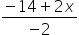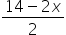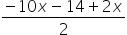Mathematics
Easy

Question

# Solve the following using the substitution method and find the value of x and y:-2x - 2y = -14-5x – y = -23

## 1, 23, 42, 14, 3Hint:

## The correct answer is: 4, 3

### Step 1 of 2:We have given two linear equations-2x – 2y = -14.....(i)-5x – y = -23......(ii)Rewrite the equation (i)-2y = -14 + 2xy==Now substitute the value of y in 2nd equation-5x -= -23= -23-8x – 14 = - 46-8x = - 46 + 14-8x = - 32x = 4Step 2 of 2:Now the value of x in 1st equation-5(4) – y =  - 23-20 – y = -23y = 3

In the substitution method, we first write one variable in the form of another variable.

### Related Questions to study#### With Turito Foundation.#### Get an Expert Advice From Turito.